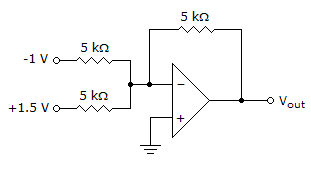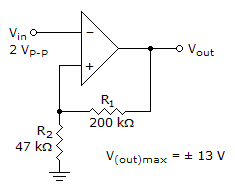# Electronic Devices - Basic Op-Amp Circuits

### Exercise :: Basic Op-Amp Circuits - General Questions

21.

Refer to the given figure. What is the output voltage?A. 0.5 V B. –0.5 V C. 2 V D. –2 V

Explanation:

No answer description available for this question. Let us discuss.

22.

What type(s) of circuit(s) use comparators?

 A. summer B. nonzero-level detector C. averaging amplifier D. summer and nonzero-level detector

Explanation:

No answer description available for this question. Let us discuss.

23.

A differentiator is used to measure

 A. the sum of the input voltages. B. the difference between two voltages. C. the area under a curve. D. the rate of change of the input voltage.

Explanation:

No answer description available for this question. Let us discuss.

24.

Refer to the given figure. Determine the lower trigger point.A. +V(out)max B. –V(out)max C. –2.47 V D. +2.47 V

Explanation:

No answer description available for this question. Let us discuss.

25.

A(n) ________ amplifier is a summing amplifier with a closed-loop gain equal to the reciprocal of the number of inputs.

 A. averaging B. scaling C. none of the above